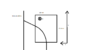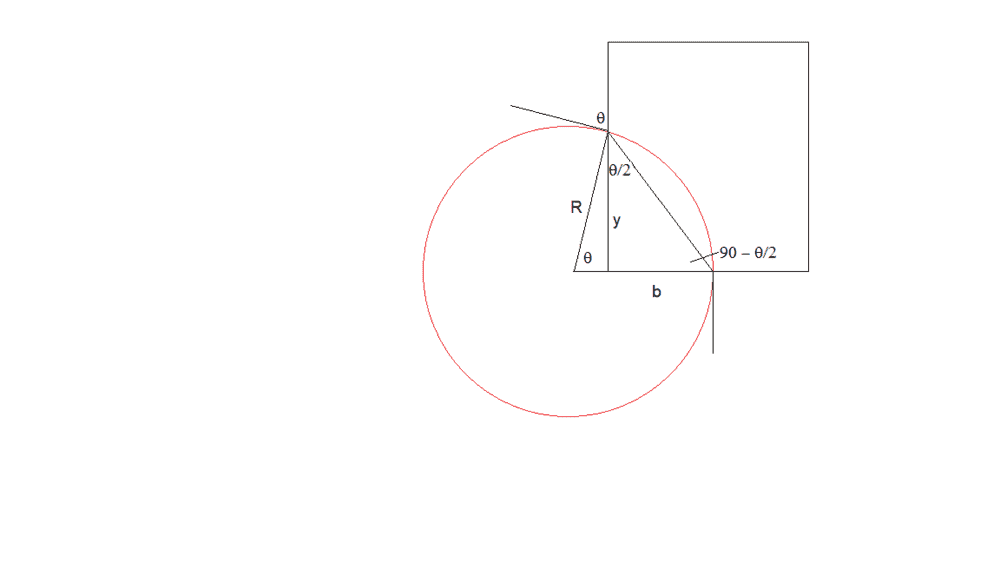# Help with the trajectory of a particle in a magnetic field

• 1Keenan

#### 1Keenan

Hello,

sorry for this stupid question but I am getting confused with equations and I need some help.
My problem is the following:
I have a dipole with known magnetic field (B=0.234T), it is 110.6 mm long and 89 mm wide (mechanical dimensions).
I want to calculate deflection of particles (electron with energy E = to 1, 2...30 MeV) and where to expect them on a detector parallel to the long edge of the quadrupole and set at a distance D.

if the detector is behind the magnet I can easily calculate its position, but the confusion I have is that in this case the detecor is on the side and I am going crazy with calculation.

I know energy, and mass, so I know curvature radius in this B=0.234T. I can assume the electrons have main axis in the middle of the magnet, so that they enter at x=0 and their x coordinate at the output would be x=44.5mm. But I am not sure on how calculate the y coordinate and then the angle. In principle if I can get y and angle I can just make it go string and I will know position on the detector... and also some of them might be reflected back if the energy is to low...
Ok, let's put it this way: how to calculatex and y coordinates at the magnet output?

I could make some quick tracking simulation but I want to solve it analitically first...

Thank you
F.

#### Attachments

•Trajectory.png
2.6 KB · Views: 84

Are you sure that your electrons will be deflected to the left in your diagram?

ok, somehow progressing!

So, trajectory are arcs of circles that are all tanget to the input velovity and their center are on the axis I made in red. This axis also contains the side of the magnet which is long b=89/2 (for example)

So if the electron has 2R<b it will be reflected back from the same entrance side.
If 2R>b but R<b it will go out from the long edge but it might not arrive on the detector if it is too short. if it has R>b but R<110.6mm it will be going out from the long edge. if R>a it will go out from the short edge on the opposite side of the manget. See attached picture

Am I correct?

Now I need to take it as geometric problem...

#### Attachments

•berkeman
@1Keenan what sources of information have you been using so far?

Looks good. Yes, once you have the radius it is a geometry problem. Draw a line from the center of the circle to the point where the particle leaves the magnetic field, that will help relating radius and the length of the arc (in radians) the particle follows.

•berkeman
@1Keenan what sources of information have you been using so far?
No source, Just thinking about the solution.
I don't want to look at a book for it, it is simple problem and it is funny to sort it out just thinking

Looks good. Yes, once you have the radius it is a geometry problem. Draw a line from the center of the circle to the point where the particle leaves the magnetic field, that will help relating radius and the length of the arc (in radians) the particle follows.
I'll do the math on Monday now it is solved. But unfortunately I think I'll have to look for the formulas, so maybe the game is over :(

but we can try to find the formula for describing magnetic field with fringes. One for electrostatic dipole exists, but I have no knowledge of something similar for magnetic dipoles

but I have no knowledge of something similar for magnetic dipoles
I'd bet that it's been done for charged particles in the Earth's Magnetic Field. That work has been going on for decades. It was used in the search for methods for predicting Radio Wave propagation. You could search for something in that direction.

but we can try to find the formula for describing magnetic field with fringes. One for electrostatic dipole exists, but I have no knowledge of something similar for magnetic dipoles
One step at a time. First the easiest field geometries.

I'd bet that it's been done for charged particles in the Earth's Magnetic Field. That work has been going on for decades. It was used in the search for methods for predicting Radio Wave propagation. You could search for something in that direction.
Well, sounds a bit too far from a magnetic dipole actually... That direction could lead to a totally wrong way

One step at a time. First the easiest field geometries.

Simplified field geometry works fine when you know effective length of the magnet.
So maybe the easiest would be to estimate the effective length of the dipole along the path of the eletron.

I mean, if input and output are opposite edges we usually consider effective lengthof the magnet. In this case input and output are on consecutive edges so maybe it would be easier to estimate effective length along the path...

Looks good. Yes, once you have the radius it is a geometry problem. Draw a line from the center of the circle to the point where the particle leaves the magnetic field, that will help relating radius and the length of the arc (in radians) the particle follows.

ok, my memories of geometry are quite bad... any hint?

See diagram below. Given b and R, can you find y and θ?
Hint: you might find useful the formula sin θ = 2t/(1+t2) where t = tan(θ/2).well, the triangle has 2 sides equal to R and the 3rd is the chord, so one angle is Theta, the other two are both equal to 90+theta/2.
I need to figure out how to find theta/2... thinking

hmm... what if:
y = R sin (theta)
and
b= y tg (theta/2)

if this is the way I can say
tg (theta/2) = b/y= b/R sin (theta) = b / R(2t/(1+t^2))

so now I have t=b / R(2t/(1+t^2))

and, if I am not making a mess with calculation, I can say that

t= +/- sqrt(b/(2R-b))

does it work?

hmmm, I'm not really sure this is the correct way...

Why not? You get expressions for the unknowns in terms of known quantities.

I don't know, I'm not really sure. I have the feeling that I made some mistake.
I did the math a few times and I get the same expression again and again... so maybe there is no mistake.

It's right. (At least, it's what I get.) What makes you think it's wrong? Put in some values for b and R, and see if you get sensible-looking answers for y and θ.

I put values and it works... but now I know why I thought it was wrong:

some particle will be reflected back (if R<b) some on the detector placed on the side (if R>b and R<110.6mm) and some would go on the front detector.
It is like in the pic I posted.

So, now these expressions for y and theta tell us what happens if all particles are going on the side... it is like my formulas at the beginning to see what happens if they go on the other side of the magnet...

I want to find something more general, to consider all the 3 cases.
I could do a small program with and if loop, but I want to do it analytically!

R < b still works. It's when R< b/2 that things change. And the other transition is not at R = a, but at y = a and θ = 2*arctan(b/a).

sure, you are right.
Diameter has to be smaller than b to have "reflection"
And actaully you expressed the second transition in the best way. If I read it correctly you mean that the output direction is the same as the diagonal, is that correct?
Anyway, here, if the detector is extremely long one can say that electron will go there anyway... this is just speculation

do you think it is possible to find an expression completely general for the 3 cases?

And actaully you expressed the second transition in the best way. If I read it correctly you mean that the output direction is the same as the diagonal, is that correct?
No. The electron emerges at the top left corner. Its path direction is not a diagonal.
do you think it is possible to find an expression completely general for the 3 cases?
No, because the boundary conditions are not continuous. I think you will need 3 separate expressions.